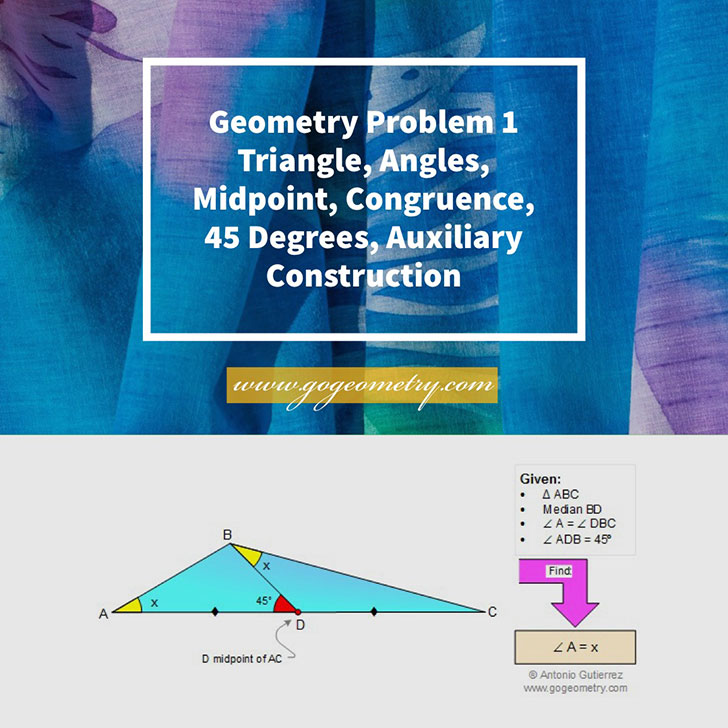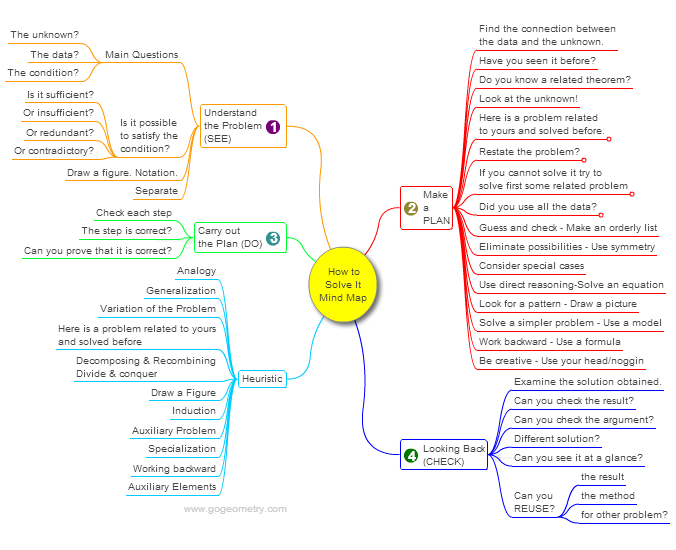# Online Geometry Problem 1: Triangle, Angles, Midpoint, Congruence, 45 Degrees, Auxiliary Construction. Level: High School, Honors Geometry, College, Mathematics Education

< PREVIOUS PROBLEM  |  NEXT PROBLEM >

In a triangle ABC, the median BD is such that the angles A and DBC are equal. If the measure of the angle ADB is 45 degrees, find the measure of angle A. Try to use elementary geometry (Euclid's Elements.)## Poster and Typography of problem 1## Geometric Art of problem 1## Mind Map: George Pólya's 1945 book, "How to Solve It"

George Pólya's 1945 book "How to Solve It, A new aspect of Mathematical Method", is a book describing methods of problem solving. It suggests the following steps when solving a mathematical problem: (1) First, you have to understand the problem. (2) After understanding, then make a plan. (3) Carry out the plan. (4) Look back on your work. How could it be better?
A mind map is a diagram used to visually organize information, knowledge, concepts or ideas.Home | Search | Geometry | Problems | All Problems | Open Problems | Visual Index | 10 Problems | Problems Art Gallery |  Art | 1-10 | Triangles | Median | Congruence | by Antonio Gutierrez View or Post a solution Last updated: Aug 5, 2018